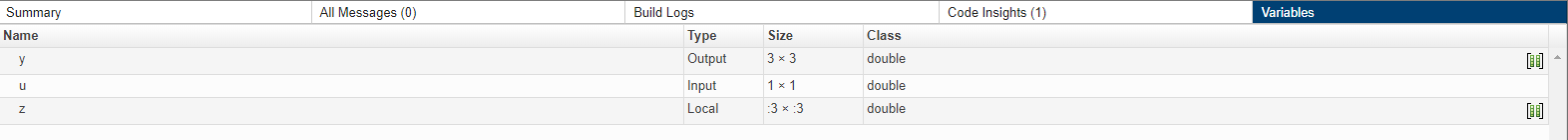## 在代码生成的可变大小支持方面与 MATLAB 的不兼容性

### 在标量扩展方面与 MATLAB 的不兼容性

```function y = scalar_exp_test_err1(u) %#codegen y = ones(3); switch u case 0 z = 0; case 1 z = 1; otherwise z = zeros(3); end y(:) = z; ``````scalar_exp_test_err1_mex(0) Subscripted assignment dimension mismatch:  ~= . Error in scalar_exp_test_err1 (line 11) y(:) = z;```

```function y = scalar_exp_test_err1(u) %#codegen y = ones(3); switch u case 0 z = 0; case 1 z = 1; otherwise z = zeros(3); end y(:) = z(1);```

### 在确定可变大小 N 维数组的大小方面与 MATLAB 的不兼容性

• 在生成的代码中返回三元素向量

• 在 MATLAB 代码中返回二元素向量

#### 解决办法

• 使用具有两个参数的 `size`

例如，`size(A,n)` 在生成的代码和 MATLAB 代码中返回相同的答案。

• 重写 `size(A)`

```B = size(A); X = B(1:ndims(A));```

此版本返回具有可变长度输出的 `X`。但是，不能将可变大小 `X` 传递给需要固定大小参数的矩阵构造函数，例如 `zeros`

### 在确定空数组的大小方面与 MATLAB 的不兼容性

```function y = foo(n) %#codegen x = []; i = 0; while (i < 10) x = [5 x]; i = i + 1; end if n > 0 x = []; end y = size(x); end```

```function out = string_size out = size(''); end```

#### 解决方法

• 重写代码以使用 `isempty` 函数，而不是 `size` 函数。

• 不要使用 `x=[]` 创建空数组，而应使用 `zeros` 创建具有特定大小的空数组。例如：

```function y = test_empty(n) %#codegen x = zeros(1,0); i=0; while (i < 10) x = [5 x]; i = i + 1; end if n > 0 x = zeros(1,0); end y=size(x); end```

### 在确定空数组的类方面与 MATLAB 的不兼容性

```function y = fun(n) x = []; if n > 1 x = ['a' x]; end y=class(x); end ```
`fun(0)` 在 MATLAB 中返回 `double`，但在生成的代码中返回 `char`。当语句 `n > 1` 为 false 时，MATLAB 不会执行 `x = ['a' x]``x` 的类为 `double`，即空数组的类。但是，代码生成器将考虑所有执行路径。它基于语句 `x = ['a' x]` 确定 `x` 的类为 `char`

#### 解决方法

```function y = fun(n) x = blanks(0); if n > 1 x = ['a' x]; end y=class(x); end```

### 在矩阵-矩阵索引方面与 MATLAB 的不兼容性

```... if isvector(A) && isvector(B) C = A(:); D = C(B(:)); else D = A(B); end ...```

### 在代码生成的矩阵索引操作方面与 MATLAB 的不兼容性

• 初始化以下样式：

```for i = 1:10 M(i) = 5; end ```

在本例中，执行循环时 `M` 的大小会改变。代码生成不支持数组大小随着时间改变而增加。

对于代码生成，需要预分配 `M`

```M = zeros(1,10); for i = 1:10 M(i) = 5; end ```

• `M(i:j)`，其中 `i``j` 在循环中改变

在代码生成过程中，不会为大小随着程序的执行而改变的表达式动态分配内存。要实现这种行为，请使用 `for-` 循环，如下所示：

```... M = ones(10,10); for i=1:10 for j = i:10 M(i,j) = 2*M(i,j); end end ...```

注意

矩阵 `M` 必须在进入循环之前定义。

### 当串联内可变大小元胞数组的花括号索引不返回任何元素时的差异

• `c` 是一个可变大小元胞数组。

• 可以使用花括号访问 `c` 的内容。例如，`c{2:4}`

• 将结果包含在串联中。例如，`[a c{2:4} b]`

• `c{I}` 不返回任何元素。`c` 为空或者花括号内的索引生成空结果。

• 类和复/实性与元胞数组的基类型相同。

• 第二个维度的大小始终为 0。

• 对于其余的维度，`Ni` 的大小取决于基类型中对应的维度是固定大小还是可变大小。

• 如果基类型中对应的维度为可变大小，则结果中的维度大小为 0。

• 如果基类型中对应的维度为固定大小，则结果中的维度也具有该大小。

• 在生成的代码中，`[...c{i}...]` 的类可能不同于 MATLAB 中的类。

`c{I}` 不返回任何元素时，MATLAB 将从串联中删除 `c{I}`。因此，`c{I}` 不影响结果的类。MATLAB 基于其余的数组并根据类的优先级确定结果的类。请参阅不同类的有效合并。在生成的代码中，由于代码生成器将 `c{I}` 视为 `[c{I}]`，因此 `[c{I}]` 的类将影响整体串联 `[...[c{I}]...]` 的结果的类。根据前面所述的规则确定 `[c{I}]` 的类。

• 在生成的代码中，`[c{I}]` 的大小可能不同于 MATLAB 中的大小。

在 MATLAB 中，串联 `[c{I}]` 为 0×0 双精度值。在生成的代码中，根据前面所述的规则确定 `[c{I}]` 的大小。

## 支持